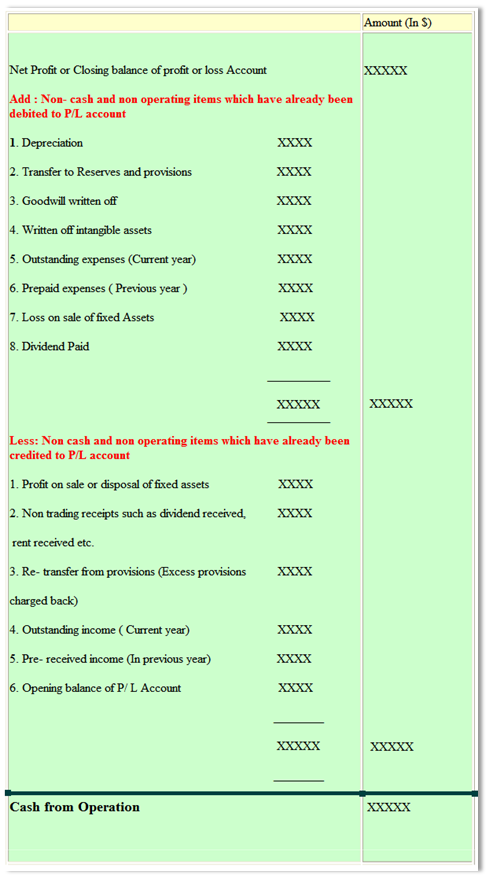222

## Latest \$type=blogging\$count=5\$author=hide\$comment=hide\$label=hide\$date=hide\$show=home

For preparing cash flow statement, we need to calculate cash from operations or cash operating profits. It is main inflow of cash which can be used for working capital. Generally, net profit of business is inflow of cash and net loss is outflow of cash. But it does not mean that cash from operation will always be equal to net profit. Net cash operating profits or loss may be less or more than net profit or loss. It may be possible that company suffered net loss but at that time, company may get net cash operating profits. Main reason of this is some non cash items which we did debit or credit in profit and loss account.

Steps to Calculate Cash from Operations :

There are two main methods of calculating cash from operation :

1st Method : Calculating Cash from operation from cash sales

If we have the information of sales, we can calculate cash from trading operations. Its formula is given below :

Cash from operation = Cash sales - ( Cash purchase + Cash operating expenses )

Or

Cash from operation = Total sales - credit sales - ( total purchase - credit purchase) - ( total expenses - non operating expenses - non cash operating expenses)

2nd Method : Calculation of cash from operation from Net profit or net loss

Under this method, we can calculate cash operating profits or loss with the help of our profit and loss account's net profit or net loss. For this, we have to make a statement for calculating of cash from operations

If you have to use second method, following points must be noted :

i) Outstanding / Accrued Expenses ( Current Year )

Outstanding expenses are those which is payable. So, there is not cash payment of such expenses. So, there is no cash outflow but it is shown in debit side of profit and loss account. That is the reason, we will add it in net profit for calculating cash operating profits. We will add only current year o/s expenses because previous year o/s expenses will be paid current year. So, there is no need to add previous year o/s expenses.

ii) Prepaid Expenses (Previous Year )

We also add prepaid expenses ( Previous year ) in net profit because it is paid in advance  in previous year and profit and loss account debited this current year.  But according to rule of cash operating profit, this prepaid expenses already was shown as outflow of cash in previous year. That is the reason, we will added it in net profit.: 1
1.i need video for this explanation plzzzzzzzzzzzzz help me

In comment, you can give your feedback, reviews, ideas for improving content or ask question relating to written content.

Name

ltr
item
Accounting Education: How to Calculate Cash from Operations
How to Calculate Cash from Operations